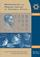Középiskolai Matematikai és Fizikai Lapok
Informatika rovattal
 Már regisztráltál? Új vendég vagy?# Angol nyelvű szám, 2002. december

Előző oldalTartalomjegyzékKövetkező oldalMEGRENDELŐLAP

## Solution of the problems for information technologyNovember 2001

I. 7. The function Factorial(N) increases very rapidly. While 5!=120, 4-byte integers are needed for storing 10!=3628800. A computer can store 100! neither as a 4-byte nor as an 8-byte integer. We know however, that every natural number has its prime factorization. For example 5!=23.3.5, or 10!=28.34 .52 .7. Your program should read the number N (1 $\displaystyle \le$N $\displaystyle \le$10000) from the keyboard, then print the prime factorization of its factorial N!

(10 points)

We solve the problem by giving two algorithms producing the same results.

First solution. Read the number. If it is 1, then the factorization is done, otherwise we begin the calculation. The first prime is 2 with exponent 1 in the beginning. Then we compute the prime factorizations of the numbers from 3 to N. The exponents from the prime factorization of the new element should be added to the exponents we already have.

Every new prime number is added to the list. (This happens if the new number is a prime number, hence the number of primes in a step can increase only by 1.)

Finally, we print all the elements in the factorization of the factorial.

We use the function PRIME to decide whether the number is prime or not. We look for divisors from 2 to the square root of the number. Because if it divides, then it is not prime, otherwise it is (its root is dropped). The first prime with exponent 1 in the beginning is 2. We go on until the prime is less than or equal to the number.

If j divides i, then the exponent is increased, otherwise, we look for the next (not greater) prime divisor.

If a prime is detected, then we have one more prime which will be j with exponent 0. (It will be 1 in the beginning of the next loop when increased.)

The last 0 exponent does not count.

Second solution. We use the Legendre's formula in the following form. If p is an arbitrary prime and N is a positive integer, then the exponent of p in the prime factorization of N! is given by ...

Of course, this is only a finite sum, since the integer parts are zero if N<pi.

The number is read. If the number is 1, then the factorization is done. The smallest prime is 2. If 2 is not greater than N, then 2 and its exponent is printed.

The next prime is 3. While the prime is not greater than the number, the prime number and its exponent is printed. Then we look for the next prime.

Computing the exponent of a prime using Legendre's formula. The exponent contains the computed sum which is 0 in the beginning.

pp contains the powers of p, which is p in the beginning. We go on until every power of p is less than or equal to N. The exponent is increased, and the next power is computed.

Determining the next prime after p. We use the function PRIME to decide whether the number is prime number. We look for divisors from 2 to the square root of the number. If it divides, then it is not prime, otherwise it is (its root is dropped).

2 steps forward can safely be done, since the only even prime is 2. This makes our program faster and more effective. Separating 2 and the subsequent primes served this purpose also. We increase the number by 2 while it is not a prime.

The Pascal programs are finding on http://www.komal.hu/verseny/2001-11/I.e.shtml.